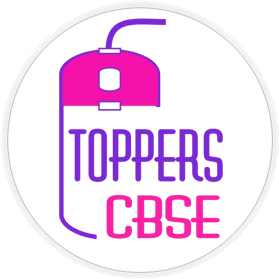• No products in the cart.

# aaaaaaaa

1. Coulomb’s force between two point charges varies with distance r between them as,$\bar{r}$,$\frac{1}{r}$,$r^{2}$,$\frac{1}{r^{2}}$,$\frac{1}{r^{2}}$
2. Force of attraction between two point charges placed in air at a distance r from each other is F. If these charges are placed in water with same separation between them, then the force of attraction between them is,$\mathrm{F}$,$80 \mathrm{~F}$,$\frac{\mathrm{F}}{80}$,$Zero$,$\frac{\mathrm{F}}{80}$,
3. Value of dielectic constant of a water,increases with increase in temperature of the water,decreases with increase in temperature of the Water,remains the same with increase in temperature of the water nothing can be said,decreases with increase in temperature of the Water
4. The order of the ratio of electrostatic force and gravitational force between a proton and an electron to,$10^{36}$,$10^{39}$ ,$10^{-36}$,$10^{-39}$,$10^{39}$
5. The unit of permittivity of free space $\epsilon_{0}$ is
,$\mathrm{C} \mathrm{N}^{2} \mathrm{~m}^{-1}$
,$\mathrm{N} \mathrm{m}^{-2} \mathrm{C}^{-2}$
,$\mathrm{C}^{2} \mathrm{~N}^{-2} \mathrm{~m}^{-2}$
,$\mathrm{C}^{2} \mathrm{~N}^{-1} \mathrm{~m}^{-2}$
,$\mathrm{C}^{2} \mathrm{~N}^{-1} \mathrm{~m}^{-2}$ ,$\left[\mathrm{e}_{0}\right]=\left[q_{1} q_{2} \mid /\left[\mathrm{F} r^{2}\right]\right.$Best Online Courses and Live Classes

#### NCERT BOOKS Class 12

• NCERT Books Class 12 PCMB
• NCERT Books Class 12 Physics
• NCERT Books Class 12 Chemistry
• NCERT Books Class 12 Biology
• NCERT Books Class 12 Maths

#### NCERT BOOKS Class 11

• NCERT Books Class 11 PCMB
• NCERT Books Class 11 Physics
• NCERT Books Class 11 Chemistry
• NCERT Books Class 11 Biology
• NCERT Books Class 11 Maths

#### NCERT Books Class 9 and 10

• NCERT Books Class 10
• NCERT Books Class 10 Science
• NCERT Books Class 10 Maths
• NCERT Books Class 9 Science
• NCERT Books Class 9 Maths

#### NCERT Books Class 6, 7, 8

• NCERT Book Class 8 Science
• NCERT Book Class 8 Maths
• NCERT Book Class 7 Science
• NCERT Book Class 7 Maths
• NCERT Book Class 6 Science
• NCERT Book Class 6 Maths

Team members of Toppers CBSE are very proud to announce that we are providing awesomeness to our learners. We think that we are going to help a lot of people to save their better future and life. We hope that we are doing best and will try to improve our resources day by day. CBSE toppers not only meant for toppers in board exams but also meant for creating class toppers. Toppers CBSE is providing Online coaching for CBSE, ICSE, State boards and Competitive exams. Toppers CBSE also contains NCERT solutions, Notes, Sample papers, Quizzes and much more. NCERT solutions for class 6th, 7th, 8th, 9th, 10th. 11th, 12th are vert awesome in pattern and languages. Revision NOTES are prepared by our best team by keeping syllabus and exam pattern in mind. ONLINE COACHING is providing by our Experienced and Dedicated team. Also we are providing online courses for easy learning at any place and any time.

hhhhhhhh